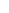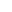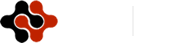International Journal of Life Science and Engineering
Articles Information
International Journal of Life Science and Engineering, Vol.1, No.3, Jul. 2015, Pub. Date: Jun. 16, 2015
Bi-Section Algorithm for Solving Linear Bi-Level Programming Problem
 Eghbal Hosseini, Department of Mathematics, Payamenur University of Tehran, Tehran, Iran.
 Isa Nakhai Kamalabadi, Department of Industry, University of Kurdistan, Sanandaj, Iran.
The bi-level programming problem (BLPP) is significant because of its application in several areas such as transportation, finance, management, computer science and so on. This problem is an appropriate tool to model these real problems. It has been proven that the general BLPP is an NP-hard problem. Therefore, the BLPP is a practical and complicated problem so solving this problem would be significant. In this paper, we attempt for developing an algorithm based on bi-section algorithm to solve BLPP. Using the Karush-Kuhn-Tucker conditions the bi-level programming problem is converted to a non-smooth single level problem, and then it is smoothed by heuristic method for using proposed bi-section algorithm. The smoothed problem is solved using bi-section algorithm which is an exact method for solving the problem. The presented approach achieves an efficient and feasible solution in an appropriate time which has been evaluated by solving test problems.
Bi-Section Algorithm, Linear Bi-Level Programming Problem, Karush-Kuhn-Tucker Conditions
 J.F. Bard, Some properties of the bi-level linear programming, Journal of Optimization Theory and Applications (1991) 68 371–378.
 L. Vicente, G. Savard, J. Judice, Descent approaches for quadratic bi-level programming, Journal of Optimization Theory and Applications (1994) 81 379–399.
 Lv. Yibing, Hu. Tiesong, Wang. Guangmin, A penalty function method Based on Kuhn–Tucker condition for solving linear bilevel programming, Applied Mathematics and Computation (2007) 1 88808–813.
 G. B. Allende, G. Still, Solving bi-level programs with the KKT-approach, Springer and Mathematical Programming Society (2012) 1 31:37 – 48.
 M. Sakava, I. Nishizaki, Y. Uemura, Interactive fuzzy programming for multilevel linear programming problem, Computers & Mathematics with Applications (1997) 36 71–86.
 S Sinha, Fuzzy programming approach to multi-level programming problems, Fuzzy Sets and Systems (2003) 136 189–202.
 S. Pramanik, T.K. Ro, Fuzzy goal programming approach to multilevel programming problems, European Journal of Operational Research (2009) 194 368–376.
 S.R. Arora, R. Gupta, Interactive fuzzy goal programming approach for bi-level programming problem, European Journal of Operational Research (2007) 176 1151–1166.
 J. Nocedal, S.J. Wright, 2005 Numerical Optimization, Springer-Verlag, New York.
 A.AL Khayyal, Minimizing a Quasi-concave Function Over a Convex Set: A Case Solvable by Lagrangian Duality, proceedings, I.E.E.E. International Conference on Systems, Man,and Cybemeties, Tucson AZ (1985) 661-663.
 R. Mathieu, L. Pittard, G. Anandalingam, Genetic algorithm based approach to bi-level Linear Programming, Operations Research (1994) 281–21.
 G. Wang, B. Jiang, K. Zhu, (2010) Global convergent algorithm for the bi-level linear fractional-linear programming based on modified convex simplex method, Journal of Systems Engineering and Electronics 239–243.
 W. T. Wend, U. P. Wen, (2000) A primal-dual interior point algorithm for solving bi-level programming problems, Asia-Pacific J. of Operational Research, 17.
 N. V. Thoai, Y. Yamamoto, A. Yoshise, (2002) Global optimization method for solving mathematical programs with linear complementary constraints, Institute of Policy and Planning Sciences, University of Tsukuba, Japan 978.
 S.R. Hejazi, A. Memariani, G. Jahanshahloo, (2002) Linear bi-level programming solution by genetic algorithm, Computers & Operations Research 29 1913–1925.
 G. Z. Wang, Wan, X. Wang, Y.Lv, Genetic algorithm based on simplex method for solving Linear-quadratic bi-level programming problem, Computers and Mathematics with Applications (2008) 56 2550–2555.
 T. X. Hu, Guo, X. Fu, Y. Lv, (2010) A neural network approach for solving linear bi-level programming problem, Knowledge-Based Systems 23 239–242.
 B. Baran Pal, D .Chakraborti, P. Biswas, (2010) A Genetic Algorithm Approach to Fuzzy Quadratic Bi-level Programming, Second International Conference on Computing, Communication and Networking Technologies.
 Z. G.Wan, Wang, B. Sun, (2012) A hybrid intelligent algorithm by combining particle Swarm optimization with chaos searching technique for solving nonlinear bi-level programming Problems, Swarm and Evolutionary Computation.
 J.F. Bard, Practical bi-level optimization: Algorithms and applications, Kluwer Academic Publishers, Dordrecht, 1998.
 J.F. Bard, Some properties of the bi-level linear programming, Journal of Optimization Theory and Applications 68 (1991) 371–378.
 S Hosseini, E & I. Nakhai Kamalabadi. Line Search and Genetic Approaches for Solving Linear Tri-level Programming Problem International Journal of Management, Accounting and Economics Vol. 1, No. 4, 2014.
 S Hosseini, E & I. Nakhai Kamalabadi. aylor Approach for Solving Non-Linear Bi-level Programming Problem ACSIJ Advances in Computer Science: an International Journal, Vol. 3, Issue 5, No.11 , September. 5.
 S Hosseini, E & I. Nakhai Kamalabadi. Two Approaches for Solving Non-linear Bi-level Programming Problem, Advances in Research Vol. 4, No.3, 2015. ISSN: 2348-0394
 J. Yan, Xuyong.L, Chongchao. H, Xianing. W, Application of particle swarm optimization based on CHKS smoothing function for solving nonlinear bi-level programming problem, Applied Mathematics and Computation 219 (2013) 4332–4339.
 Xu, P, & L. Wang. An exact algorithm for the bilevel mixed integer linear programming problem under three simplifying assumptions, Computers & Operations Research, Volume 41, January, Pages 309-318 (2014).
 Wan, Z, L. Mao, & G. Wang. Estimation of distribution algorithm for a class of nonlinear bilevel programming problems, Information Sciences, Volume 256, 20 January, Pages 184-196(2014).
 Zheng, Y, J. Liu, & Z. Wan. Interactive fuzzy decision making method for solving bi-level programming problem, Applied Mathematical Modelling, Volume 38, Issue 13, 1 July, Pages 3136-3141(2014).
 Zhang, G, J. Lu, J. Montero, & Y. Zeng, Model. solution concept, and Kth-best algorithm for linear tri-level, programming Information Sciences 180 481–492 (2010) .
 A. Silverman. Richard, Calculus with analytic geometry, ISBN: 978-964-311-008-6, 2000.
 Y. Zheng, J. Liu, Z. Wan, Interactive fuzzy decision making method for solving bi-level programming problem, Applied Mathematical Modelling, Volume 38, Issue 13, 1 July 2014, Pages 3136-3141.
 Y. Jiang, X. Li, C. Huang, X. Wu, An augmented Lagrangian multiplier method based on a CHKS smoothing function for solving nonlinear bi-level programming problems, Knowledge-Based Systems, Volume 55, January 2014, Pages 9-14.
 X. He, C. Li, T. Huang, C. Li, Neural network for solving convex quadratic bilevel programming problems, Neural Networks, Volume 51, March 2014, Pages 17-25.
 Z. Wan, L. Mao, G. Wang, Estimation of distribution algorithm for a class of nonlinear bilevel programming problems, Information Sciences, Volume 256, 20 January 2014, Pages 184-196.
 P. Xu, L. Wang, An exact algorithm for the bilevel mixed integer linear programming problem under three simplifying assumptions, Computers & Operations Research, Volume 41, January 2014, Pages 309-318.
 E. Hosseini, I. Nakhai Kamalabadi, A Genetic Approach for Solving Bi-Level Programming Problems, Advanced Modeling and Optimization, Volume 15, Number 3, 2013.
 E. Hosseini, I. Nakhai Kamalabadi, Solving Linear-Quadratic Bi-Level Programming and Linear-Fractional Bi-Level Programming Problems Using Genetic Based Algorithm, Applied Mathematics and Computational Intelligence, Volume 2, 2013.
 E. Hosseini, I.Nakhai Kamalabadi, Taylor Approach for Solving Non-Linear Bi-level Programming Problem ACSIJ Advances in Computer Science: an International Journal, Vol. 3, Issue 5, No.11, September 2014.
 E. Hosseini, I.Nakhai Kamalabadi, Solving Linear Bi-level Programming Problem Using Two New Approaches Based on Line Search International Journal of Management sciences and Education, Vol. 2, Issue 6, 2014, 243-252.
 E. Hosseini, I.Nakhai Kamalabadi, Two Approaches for Solving Non-linear Bi-level Programming Problem, Advances in Research, 3(5), 2015.
 Froberg, carl-Erik. Numerical Mathematics, Theory and Computer Applications. The Benjamin Publishing Company, 1985.
 Hamming, R.W. numerical methods for scientists and engineers. Mc Graw-Hill, New York, 1962.
 Walter Rudin, Principles of Mathematical Analysis, 3rd ed. McGraw-Hill, New York, 1976.ISSN Print: 2381-697X
ISSN Online: 2381-6988
Current Issue:
Vol. 3, Issue 4, December Submit a Manuscript Join Editorial Board Join Reviewer Team
 About This Journal All Issues Open Access Indexing Payment Information Author Guidelines Review Process Publication Ethics Editorial Board Peer Reviewers600 ATLANTIC AVE, BOSTON,
MA 02210, USA+001-6179630233
JournalsJournal ListsAuthor GuidelinesPayment InformationSubmit a ManuscriptJoin as Editorial MembersJoin as ReviewersPrivacy NoticeTerms of ConditionsHelp Center